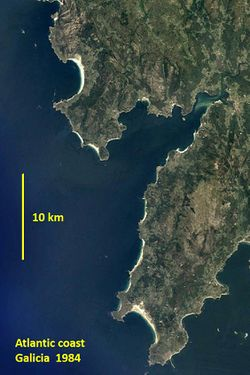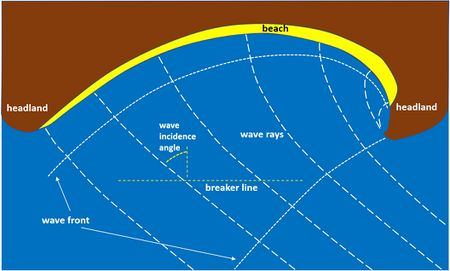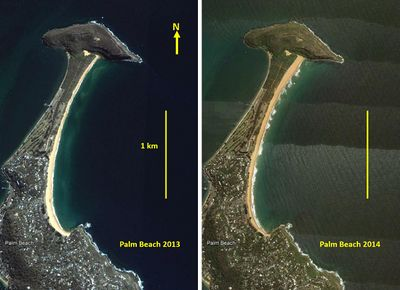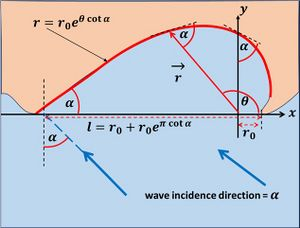# Embayed beaches

(diff) ← Older revision | Latest revision (diff) | Newer revision → (diff)Fig. 1. The rocky shore of western Galicia (Spain) with numerous embayed beaches. Google Earth image.

## Introduction

Many rocky coasts host small beaches that are semi-enclosed between headlands (natural capes or promontories), see Fig. 1. They are also called 'pocket beaches' or 'headland-bay beaches'. Small sheltered embayed beaches are called coves. If access from the land side is possible, natural embayed beaches are highly valued by tourists because of the spectacular landscape. This is less the case when they are created artificially by isolating a linear beach between long groins.

Embayed beaches in general do not receive much sediment from the hinterland. Beach sediment is mainly derived from local sources (cliff erosion, for instance); therefore no strong correlation exists between grainsize and wave intensity. For sufficiently great headland length (at least 150 m) the headlands block longshore transport and thus sediment exchange with adjacent coastal stretches. Only under severe storm conditions can sediment pass the headlands. The beach exchanges sand with the shoreface, depending on fluctuations in the wave climate (high-energy storm waves or low-energy swell). The presence of a beach implies that net offshore loss of sediment is limited (otherwise no beach would be present). Hence, the budget of the sand body comprised between the headlands is virtually closed.

## Shoreline fluctuation and beach rotation

The plan shape of embayed beaches depends on the way in which the sand body is distributed in cross-shore and longshore direction. The shoreline orientation depends, more than for non-embayed beaches, on the offshore wave incidence direction, on wave refraction and on wave diffraction. The wave incidence angle changes when waves approach the shore. The wave front becomes more aligned with the shoreline, due to refraction over the depth contours and due to diffraction at the headlands. The most representative value of the wave incidence angle for estimating the littoral drift is just landward from the breaker line where longshore transport is greatest (Fig. 2). The wave incidence angle, together with the breaker-induced wave energy dissipation, determine the sediment transport gradients along the beach (see Shallow-water wave theory and Littoral drift and shoreline modelling for a more detailed explanation).Fig. 2. Schematic representation of the theoretical equilibrium planform of an embayed beach of the logspiral type.Fig. 3. Example of beach rotation, Palm Beach, Australian east coast. In 2013 the beach width was greatest in the southern part; in 2014 the width was greatest in the northern part. Images Google Earth.

Because the longshore drift always vanishes at the headlands, a strong gradient in sand transport along the beach arises when the average direction of the incident waves shifts from a perpendicular to an oblique angle with the shoreline. Sand transport is greatest at the location where the angle is most oblique; the gradient is positive (transport divergence) on the updrift side of this location and negative (transport convergence) on the downdrift side. The resulting sand redistribution induces shoreline rotation; the updrift side retreats and the downdrift side progrades (Fig. 3). Accretion and erosion can be significant in response to seasonal changes in the mean wave direction. Significant accretion and erosion can also occur over shorter periods with high wave energy. A directionally fluctuating wave climate can lead to substantial beach rotation events from the timescale of storm duration to the decadal timescale.

The mean shoreline orientation near the downdrift headland (the headland toward which waves are directed) is approximately perpendicular to the mean wave direction, to satisfy the zero drift condition. The shoreline is curved toward the updrift headland, as a result of wave diffraction at this headland. When the directional spread of incident waves is small, strong wave diffraction leads to a strongly curved shoreline (Fig. 2). For greater directional spread the shoreline curvature is less since refraction dominates over diffraction.

Rotation is not the only mode of shoreline change. Shoreline advance and retreat can also be due to seasonal or storm-induced fluctuations in wave energy and wave period that redistribute sand between beach and shoreface, similar as for non-embayed beaches, see Shoreface profile. Cross-shore processes may also induce the formation of longshore bars and rip cells. Strong rip currents can occur near the headlands; rips associated with gaps between nearshore sandbars often occur on wide embayed beaches, see Rhythmic shoreline features. Fluctuations in the shoreline position are generally more strongly related to cross-shore sand redistribution processes than to longshore sand redistribution and associated shoreline rotation.

Another phenomenon observed on some embayed beaches (particularly steep coarse-grained- reflective-type beaches under close to shore-normal wave incidence) is the reflection of edge waves off the headlands. The resulting standing wave pattern stimulates the development of beach cusps.

## Beach rotation modelling

Simulation of the morphological adaptation of embayed beaches to changes in the wave climate requires a two-dimensional morphodynamic process model, if not only beach rotation but also other cross-shore and longshore sediment exchange processes play a role. These models can deal with complex topography and wave spectrum but are costly for the simulation of long-term processes such as the evolution towards equilibrium. If beach rotation is the main subject of interest, simpler models can be used, provided data is available for calibration of the model parameters. A model with good performance is based on the following assumptions

• during each short time interval the wave climate can be characterized by a significant wave height $H_s$, peak wave period $T_p$ and wave incidence angle $\theta$;
• for each wave angle $\theta$ the corresponding equilibrium shoreline angle $\phi_{eq}$ is given by the linear relationship, $\phi_{eq} = a \theta + b$, where $a, b$ are independent of $\theta, H_s, T_p$;
• the relaxation time $T$ is inversely proportional to the wave power, $T=c / P, \; P= T_p \, H_s^2$.

The shoreline orientation $\phi$ at time $t$ can then be computed from the equation

$\Large\frac{d \phi}{dt}\normalsize = \Large\frac{1}{T}\normalsize (\phi_{eq}-\phi) . \qquad (1)$

The values of the parameters $a, b, c$ can be obtained through fitting Eq. (1) to data for a sufficiently long period in which shoreline orientation observations are available.

## Equilibrium planformFig. 4. Sketch and symbols for the logspiral plan shape formula, assuming equilibrium and ignoring wave refraction outside the embayment.

The shoreline will reach an equilibrium shape only under exceptionally stable conditions, as waves are generally refracted over slowly adapting depth contours on the less active coastal zone. Hence, the shoreline is almost always in a transitional state, continuously adapting to changes in wave direction and intensity. Even if equilibrium conditions are not well met, the plan shape of embayed beaches has a characteristic form when oblique wave incidence prevails. This shape is sketched in Fig. 2 and visible in the example shown in Fig. 3. Embayed beaches under influence of wave diffraction (not too wide, not too far protruding headlands) can be parameterized with the empirical formula

$r = r_0 \exp(\theta \cot \alpha) , \qquad (2)$

where $r$ is the length of a vector $\vec{r}$ with components $(x(\theta)=r \cos \theta, y(\theta)=r \sin \theta)$, see Fig. 4 for definitions. The theoretical diffraction point is situated at $x=0, y=0$ and the tip of the headland at $x=r_0, y=0$. The parameter $\alpha$ is the angle between the vector $\vec{r}$ and the tangent to the curve defined by Eq. (2), because $dy/dx=\tan(\theta + \alpha)$. In the case of morphological equilibrium, the parameter $\alpha$ equals the wave incidence direction relative to the bay orientation if refraction outside the embayment can be ignored. The parameter $r_0$ can be derived from the embayment length $l$ as shown in Fig. 4. The logspiral shape can be justified for simple topographies and has been applied with reasonable success to many headland bay beaches shaped under influence of wave diffraction, i.e., for wave incidence angles larger than 40o . However, the application to more complex topographies is questionable, for example if headlands protrude far offshore or if wave rays are refracted by offshore rock outcrops.

More recent studies advocate a parabolic representation of the equilibrium plan shape,

$r = r_0 + r_1 \theta^{-1} + r_2 \theta^{-2} . \qquad (3)$

The angle $\theta$ is the angle between the wave crest line from the diffraction point (taken as the $x$-axis) and the vector $\vec{r}$ with components $(x(\theta)=r\cos\theta, y(\theta)=r \sin\theta)$, see Fig. 5. The form (3) applies to the curved updrift part of the headland-bay beach under influence of wave diffraction. It has three parameters instead of two; it is therefore more flexible than the form (2) and can accommodate more symmetrical plan forms. More symmetrical plan forms are typical for bays with far protruding headlands. The parameters $r_0, r_1, r_2$ can be derived from characteristics of the headland configuration and the wave incidence direction. However, there is no general prescription with which these parameters can be determined unambiguously; accurate determination requires least square fitting to the long-term average plan shape.